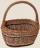# Nineteenth member

Find the nineteenth member of the arithmetic sequence:

a1=33
d=5
find a19

Result

a19 =  123

#### Solution:Leave us a comment of example and its solution (i.e. if it is still somewhat unclear...):

Showing 1 comment:Deas
This app helps so much## Next similar examples:

1. Sequence 3Write the first 5 members of an arithmetic sequence: a4=-35, a11=-105.
2. SequenceBetween numbers 1 and 53 insert n members of the arithmetic sequence that its sum is 702.
3. AS sequenceIn an arithmetic sequence is given the difference d = -3 and a71 = 455. a) Determine the value of a62 b) Determine the sum of 71 members.
4. Sequence 2Write the first 5 members of an arithmetic sequence a11=-14, d=-1
5. SequenceWrite the first 6 members of these sequence: a1 = 5 a2 = 7 an+2 = an+1 +2 an
6. SequenceWrite the first 7 members of an arithmetic sequence: a1=-3, d=6.
7. 6 termsFind the first six terms of the sequence. a1 = 7, an = an-1 + 6
8. Sum of membersWhat is the sum of the first two members of the aritmetic progression if d = -4.3 and a3 = 7.5?
9. Functions f,gFind g(1) if g(x) = 3x - x2 Find f(5) if f(x) = x + 1/2
10. TreesA certain species of tree grows an average of 0.5 cm per week. Write an equation for the sequence that represents the weekly height of this tree in centimeters if the measurements begin when the tree is 200 centimeters tall.
11. AP - simpleDetermine the first nine elements of sequence if a10 = -1 and d = 4
12. SeatsSeats in the sport hall are organized so that each subsequent row has five more seats. First has 10 seats. How many seats are: a) in the eighth row b) in the eighteenth row
13. Apples in basketsDetermine how many apples are in baskets when in the first basket are 4 apples, and in any other is 29 apples more than the previous, and we have eight baskets.
14. One halfOne half of ? is: ?
15. Factory and divisionsThe factory consists of three auxiliary divisions total 2,406 employees. The second division has 76 employees less than 1st division and 3rd division has 212 employees more than the 2nd. How many employees has each division?
16. Theorem proveWe want to prove the sentense: If the natural number n is divisible by six, then n is divisible by three. From what assumption we started?
17. Volleyball8 girls wants to play volleyball against boys. On the field at one time can be six players per team. How many initial teams of this girls may trainer to choose?